# Two infinitely long parallel wires carry current in -k direction the wire that intersect xy plane...

Two infinitely long parallel wires carry current in -k direction the wire that intersect xy plane on the x-axis at x= -3m carries 20A of current. The second wire intersects xy plan at x=+3m and carries 40A. Find the y component of the total magnetic field on y axis at y =4

Find the force per meter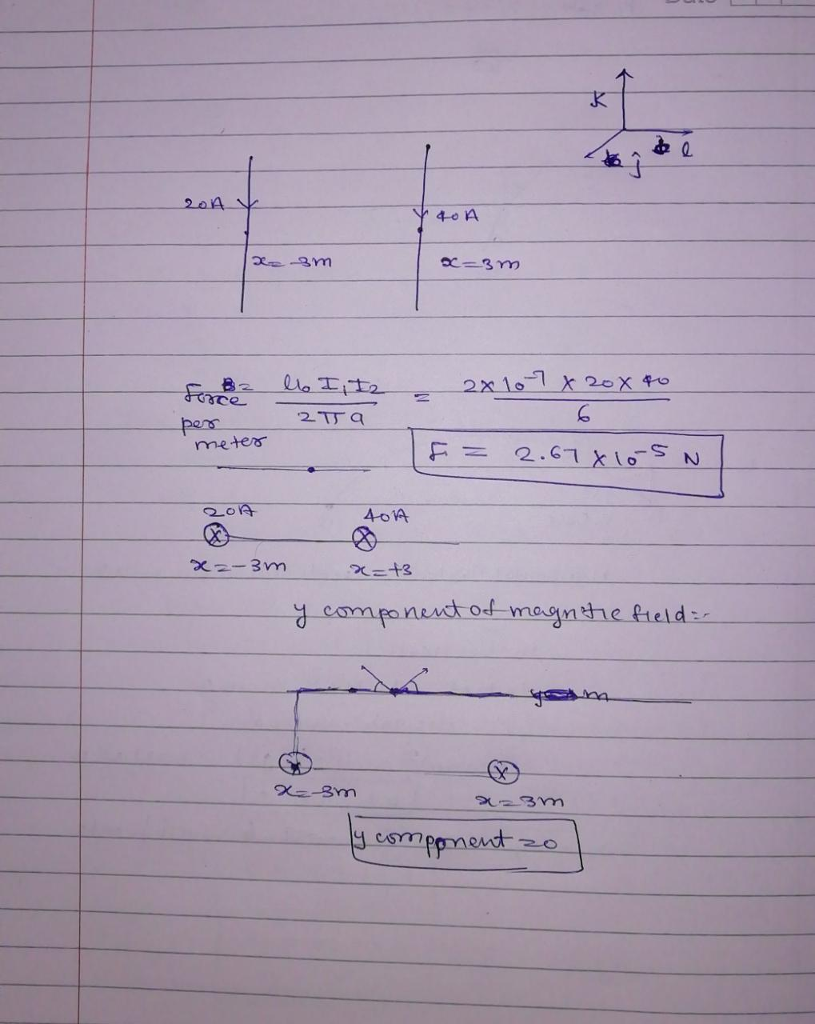#### Earn Coin

Coins can be redeemed for fabulous gifts.

Similar Homework Help Questions
• ### PLEASE HELP!!! 8. Two long, parallel wires each carry a current I in the same direction...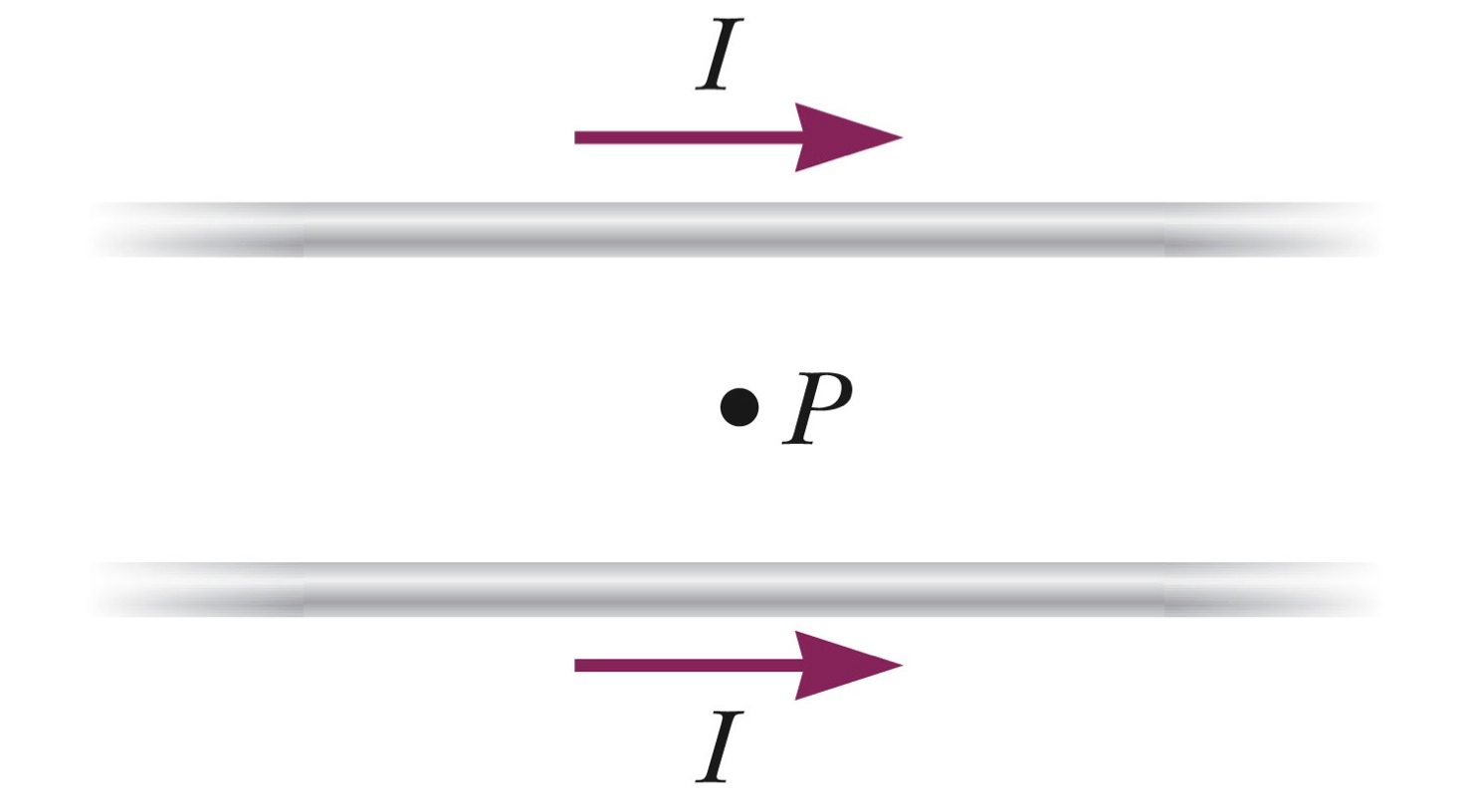PLEASE HELP!!! 8. Two long, parallel wires each carry a current I in the same direction as shown in the figure. The magnetic field at the point P, midway between the two wires is ________. zero directed into the page directed out of the page parallel to the wires in the same direction as the current. parallel to the wires, in the opposite direction as the current. 9. A long, straight wire carries a current of 20A to the right....

• ### Question 20 (7 points) Two long straight wires carry currents perpendicular to the xy-plane. One wire...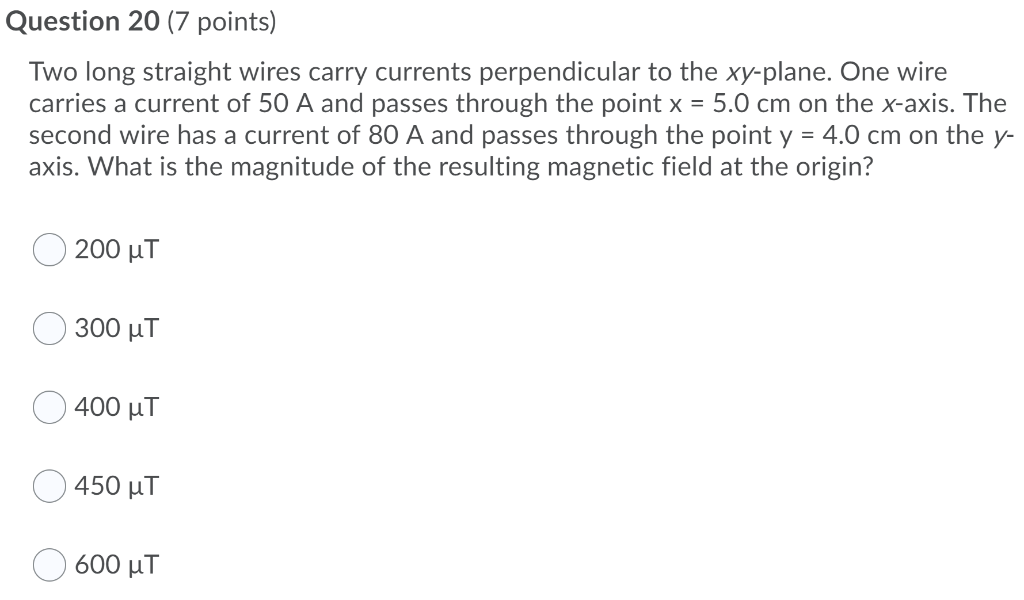Question 20 (7 points) Two long straight wires carry currents perpendicular to the xy-plane. One wire carries a current of 50 A and passes through the point x = 5.0 cm on the x-axis. The second wire has a current of 80 A and passes through the point y = 4.0 cm on the y- axis. What is the magnitude of the resulting magnetic field at the origin? 200 ut 300 uT 400 uT 450 uT 600 uT

• ### Four long parallel wires each carry a 2.0 A current in the same direction. The wires...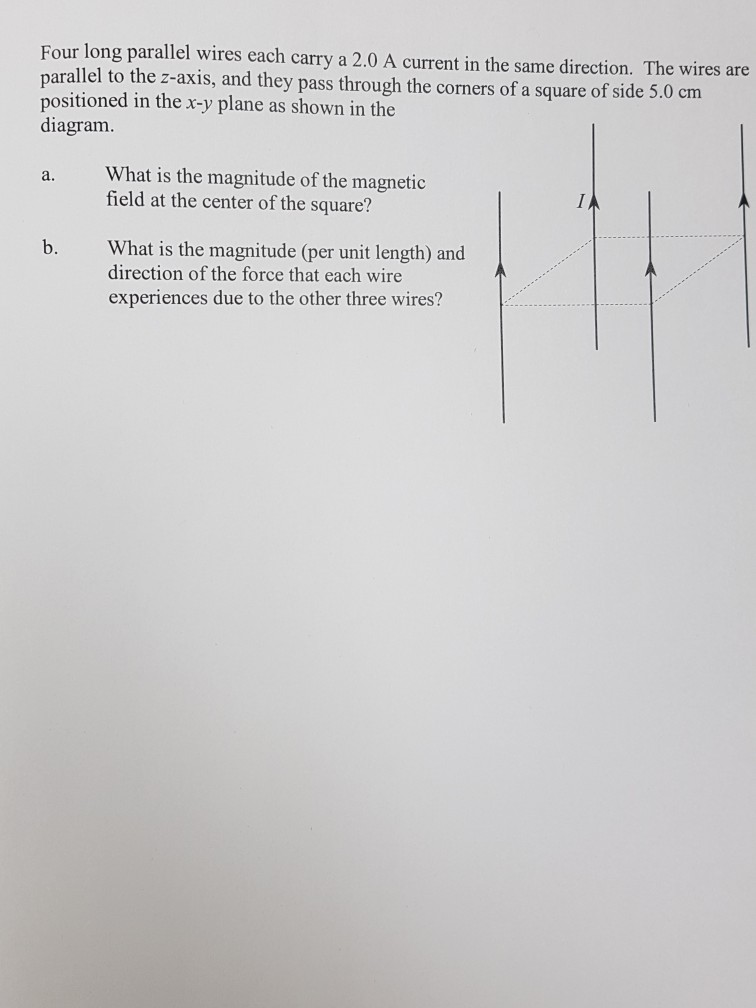Four long parallel wires each carry a 2.0 A current in the same direction. The wires are parallel to the z-axis, and they pass through the corners of a square of side 5.0 cm in the x-y plane as shown in the diagram. What is the magnitude of the magnetic field at the center of the square? a. b. What is the magnitude (per unit length) and direction of the force that each wire experiences due to the other three...

• ### A long wire carrying a 4.6A current perpendicular to the xy-plane intersects the x-axis at x=-1.6cm....

A long wire carrying a 4.6A current perpendicular to the xy-plane intersects the x-axis at x=-1.6cm. A second, parallel wire carrying a 2.8A current intersects at x=+1.6cm. Part A At what point on the x-axis is the magnetic field zero if the two currents are in the same direction? Part B At what point on the x-axis is the magnetic field zero if the two currents are in opposite directions?

• ### Two infinitely long, straight wires are parallel and separated by a distance of one meter. They...

Two infinitely long, straight wires are parallel and separated by a distance of one meter. They carry currents in the same direction. Wire 1 carries 6 times thecurrent as wire 2 does. Determine the perpendicular distance from wire 1 to a point where the net magnetic field is zero between the two wires.

• ### Two Wires Points: 1 Consider two parallel conducting wires along the direction of the Z axis...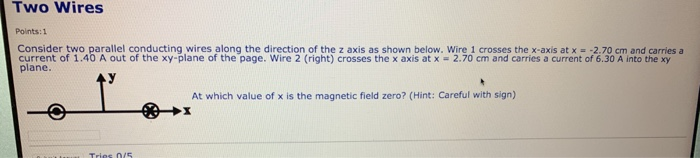Two Wires Points: 1 Consider two parallel conducting wires along the direction of the Z axis as shown below. Wire 1 crosses the x-axis at x = -2.70 cm and carries a current of 1.40 A out of the xy-plane of the page. Wire 2 (right) crosses the x axis at x = 2.70 cm and carries a current of 6.30 A into the xy plane. At which value of x is the magnetic field zero? (Hint: Careful with sign)...

• ### 1. Two infinitely long parallel wires are situated at x = +1 meter along the x-axis...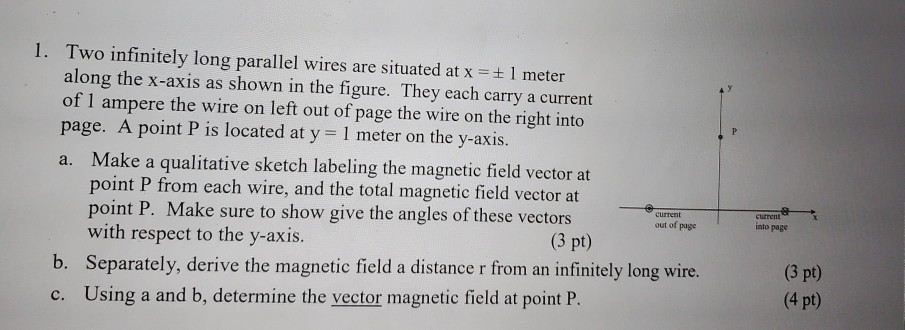1. Two infinitely long parallel wires are situated at x = +1 meter along the x-axis as shown in the figure. They each carry a current of 1 ampere the wire on left out of page the wire on the right into page. A point P is located at y = 1 meter on the y-axis. a. Make a qualitative sketch labeling the magnetic field vector at point P from each wire, and the total magnetic field vector at point...

• ### Magnetic Fields: Two long, straight wires in the xy plane and parallel to the x axis find the...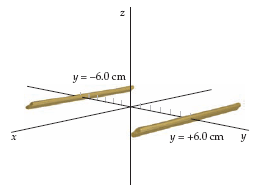The figure below shows two long, straight wires in the xy plane and parallel to the x axis. One wire isat y = −6.0 cm and the other wire is at y = +6.0 cm. The current in each wire is 34 A. Ifthe currents are both in the −x direction, find the magnetic field at the following points on the y axis.(a) y = −1.0 cm________________ µT (b) y = 0_______0_____ µT (c) y = +1.0 cm________________ µT (d)...

• ### A long wire carrying a 5.0 A current perpendicular to the xy-plane intersects the x-axis at x =...

A long wire carrying a 5.0 A current perpendicular to the xy-plane intersects the x-axis at x = -2.00 cm. A second parallel wire carrying a 3.00 A current intersectsthe x-axis at x = +2.00 cm. At what point or points on the x-axis is the magnetic field zero (a) if the two currents are in the same direction and (b) if the twocurrents are in opposite directions?

• ### Question Completion Status: QUESTION 2 Two infinitely long parallel wires are separated by distance d =...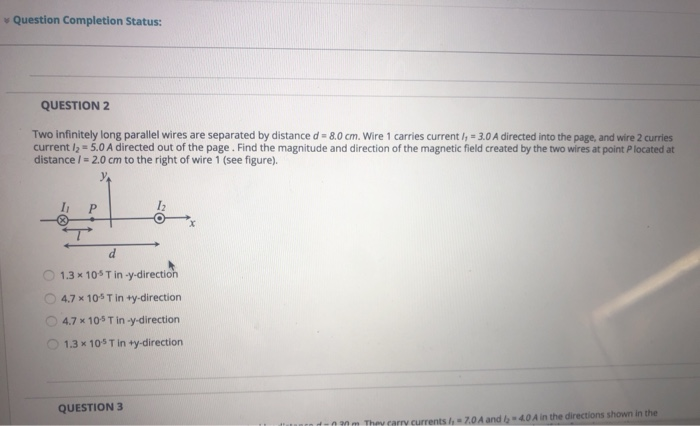Question Completion Status: QUESTION 2 Two infinitely long parallel wires are separated by distance d = 8.0 cm. Wire 1 carries currently - 3.0 A directed into the page, and wire 2 curries currently = 5.0 A directed out of the page. Find the magnitude and direction of the magnetic field created by the two wires at point P located at distance/= 2.0 cm to the right of wire 1 (see figure). IP 1.3 x 105 Tiny-direction 4.7 * 105...